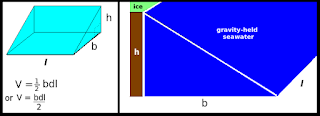## Sunday, January 17, 2016

### The Gravity of Sea Level Change - 4Fig. 1 Basic formula @ Math Teacher adapted
In the previous post of this series estimates were made based upon formulas tied to the prism shape (The Gravity of Sea Level Change - 3).

Using the prism shape, I calculated the cubic kilometers of sea water held by ice sheet gravity around Greenland and Antarctica.

The volume values were not taken further to determine how much sea level rise (SLR) would take place.

So, in today's post I will do that calculation while using a simpler, more direct formula by replacing the conceptual shape from a prism to a wedge (Fig. 1).

The simple formula then becomes V = bhl / 2,  where "b" is the distance from the coastline to the "hinge point" (The Evolution and Migration of Sea Level Hinge Points); "h" is the depth of the sea level fall (SLF) to be released by ice mass / gravity loss, and "l" is the length of the coastline.

Using the figures from the previous post, first we begin with the 1m global mean average SLR problem:
the unequal raw numbers are:

b = 2000 km
h = 20 m
l = 44,087 km (Greenland coastline length)
l = 17,968 km (Antarctica coastline length)

(see Wikipedia for those coastline lengths)

converting all figures to meters, the preliminary figures are:

b = 2000 km * 1000 = 2,000,000 m
l = 44,087 km * 1000 = 44,087,000 m (Greenland)
l = 17,968 km * 1000 = 17,968,000 m (Antarctica)
l = 62,055,000 m (sum of lengths of both coastlines)

Those figures fit the formula as follows:

V = (b * h * l) / 2
V = (2,000,000 m * 20 m * 62,055,000 m) / 2
V = (2.4822 × 10¹⁵ m3) / 2 (meters)
V = (2.4822 × 10¹² km3) / 2 (kilometers)
V = 1.2411 × 10¹² km3

The next thing to do is figure out how much SLR is generated by this seawater once it is relocated:
2.78 x 10-6 m = 0.00000278 m (1 km3 = 2.78 x 10-6 m of SLR)
(for mm << m we divide by 1000, to derive 0.00000000278 mm)
thus: 1.2411 × 10¹² km3 × 0.00000000278 mm = 3,450.258 mm
3,450.258 mm = 11.32 ft. (divide mm by 304.8 to get ft.)
(Climate Sanity). This is 11.32 feet of gravity induced SLR added onto the 1 meter of global mean average SLR generated from the ice sheet melt water of Greenland, and the additional 1 meter of global mean average SLR (2 m of global mean average SLR total) from Antarctica/Greenland melt water / calving.

Let's recap: Professor Mitrovica calculated that a 20 meter drop in sea level (SLF) around Greenland, extending out 2,000 km to the "hinge line," would take place if enough Greenland ice sheet melted to raise the global mean average sea level by 1 meter (I added another meter --from Antarctica -- so 2 m total).Fig. 2  Blue area relocated to Orange area

Thus, in addition to the 2 m (6.56 ft.) from the ice melt, there is an additional 11.32 feet of SLR (6.56 + 11.32 = 17.88 feet).

And don't forget the 20 m of SLF @ Greenland and Antarctica is also SLC.

The additional seawater distribution is not exclusively associated with direct ice melt in the sense that it is already seawater, because that additional distribution is due to gravitational power lost when the ice sheet on land melts.

The ice sheet incrementally loses gravity in proportion to mass loss (Newton).

That 1.2411×10¹² km3 of gravity-held water is released from the coastal areas, and is then relocated to various spots around the global ocean (Fig. 2).

This happens according to the Earth's rotational, gravitational, etc., forces acting upon that released seawater (adding an additional 11.32 ft of SLR in places).

Can you see that I had a valid reason for hypothesizing that this "ghost water" is probably missing from consideration in the traditional SLC software models (The Evolution of Models - 19) which have repeatedly underestimated SLR?

I have mentioned that I also think it is a reason that words like "El Nino, King 'Tides', coastal 'flooding', etc." are conjured up by some influential meteorologists (they are missing some obvious factors).

Maybe next time we will take a look at the impact a 100 m SLF (a la Professor Mitrovica) around Greenland and Antarctica would have.

The next post in this series is here, the previous post in this series is here.

1.The 'ghost water'sounds about right ...

2.Hey Dredd, great job with this calculation. Makes it "real."

Tom

3.something else may (also) be a big problem with melting ice sheets:

Massive reservoir of greenhouse gas may be hiding in ice sheets

http://www.ibtimes.com.au/massive-reservoir-greenhouse-gas-may-be-hiding-ice-sheets-1500454

Ice sheets may contain gas hydrate stability zone that could serve as a reservoir containing a huge amount of natural greenhouse gases, according to a research published in Nature Communications. This new study shows that significant amount of methane may have been released into the atmosphere from 22,000 years ago and it could occur soon when ice sheets melt away completely. [more]

Tom

1.Tom,

Yes, the billions of small bubbles indicate that a lot of it was in the area.

Also, when the sea level drops 20-100 meters the clathrates will rains hell.

2.The article says "it could occur soon when ice sheets melt away completely" ... when the ice fractures any trapped pockets of gas can be released via those cracks prior to complete melt and disintegration IMO.